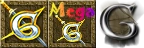This article documents Glest, GAE, and MegaGlest This is noted in the colored backgrounds, which denote lines as being engine specific. See the key below.[Editing help]
v  d  e

The menu XML defines how the menu screen works. It controls the fog, rain, and water level, as well as the camera positions and angles for each menu option. It varies slightly in the differing versions of Glest, since the forks add different menu options, such as GAE's save menu and MegaGlest's modified introduction.

XML

GAE Only
GAE and MegaGlest Only
<?xml version="1.0"?>
<water value="true" height="3" />
<rain value="true" />
<fog value="true" density="0.025" />
<camera>
<start-position x="5" y="10" z="40" />
<root-position x="-9" y="11" z="10" />
<config-position x="4" y="17" z="0" />
<info-position x="5" y="6" z="-5" />
<new-game-position x="12" y="17" z="5" />
<join-game-position x="14" y="15" z="5" />
<scenario-position x="10" y="13" z="2" />
<!-- Start GAE Only -->
<!-- End GAE Only -->
<start-rotation x="0" y="0" z="0" />
<root-rotation x="0" y="-40" z="0" />
<config-rotation x="0" y="-5" z="0" />
<info-rotation x="0" y="25" z="0" />
<new-game-rotation x="0" y="10" z="0" />
<join-game-rotation x="0" y="12" z="0" />
<scenario-rotation x="0" y="-10" z="0" />
<!-- Start GAE Only -->
<!-- End GAE Only -->
</camera>
<!-- Start GAE Only -->
<logos>
<total-conversion value="false" />
<gae-logo value="false" />
<gpl-logo value="true" />
</logos>
<!-- End GAE Only -->
<!-- Start MG Only -->
<intro>
<intro-time value="47500" />
<appear-time value="1000" />
<show-time value="1500" />
<disappear-time value="1000" />
<show-intro-pictures value="8" random="true" time="2500" />
<show-intro-models value="true" random="true" min-anim-speed="0.010" max-anim-speed="0.6" />
<camera>
<start-position x="6" y="8.5" z="11" />
<start-rotation x="0" y="-80" z="0" />
</camera>
</intro>
<!-- End MG Only -->

Documentation

water

Toggles and sets the height value, in game tiles, of the water. Each game tile is equal to roughly 1 tile in Blender. If the water is turned off, exclude the height value:

<water value="false" />

rain

Toggles on or off rain. There is not yet a method of adding snow.

fog

Toggles and sets the density of fog. The density is the thickness of the fog. You cannot yet choose the color of this fog, and it will be rendered the standard grey.

camera

These settings control the position and rotation of the camera for each menu. Positions are where the camera is located in space (in Glest tile units, where one tile is about the same as one tile in Blender, centered to the center of the model), while rotations are degrees in a direction from that position. Because there is no easy way to find out positions and rotations, modifying this is generally an action of trial and error.

All values are stored as x, y, and z. X is the horizontal axis, z is the depth axis, and y is the vertical axis.

Element name Details
start-position Sets the very first position the camera will be in when the game starts. It will not stay in this position and will move towards the root menu position, so this is entirely a cinematic effect.
root-position The camera location for the main menu.
config-position Position for the configuration (options) screen.
info-position Position for the info screen (subscreen in the options menu).
new-game-position Position for the new game screen.
join-game-position Position for the join game screen.
start-rotation The direction the camera is facing when the program starts, before it moves for the main menu position.
root-rotation The camera rotation at the main menu.
config-rotation The camera rotation at the configuration (options) screen.
info-rotation The camera rotation at the info screen (subscreen in the options menu).
new-game-rotation The camera rotation at the new game screen.
join-game-rotation The camera rotation at the join game screen.

total-conversion

A GAE only value that defines whether or not the mod is meant to be a regular mod or a complete conversion. Complete conversions will hide the version numbers and show a different logo at the side.

gae-logo

If true, an alternative GAE logo will also be shown at the bottom of the main menu. Good for full conversion mods because you can then change the regular logo.

gpl-logo

If true, the GPL logo will be shown at the bottom of the main menu.

intro

A MegaGlest only tag, these settings control the game intro. Modifying this is generally an action of trial and error. All time values are in milliseconds (1 second = 1000 milliseonds).

Element name Details
intro-time This is the total amount of time that the intro should display before showing the start menu.
appear-time This is the amount of time that it will take one line of intro data to fade into display.
show-time This is the amount of time that it will take one line of intro data to remain in display after fading in.
disappear-time This is the amount of time that it will take one line of intro data to fade out of display.
menu-intro-music The name of the intro music file to play (located in menu-music-path).
show-intro-pictures This node describes how intro pictures should display.

value= number of images to display (using the following path and naming: data/core/menu/textures/intro*.*).
random = true or false meaning display order of images should be sequential (false) or random (true).
time = time in milliseconds to disply the picture.

show-intro-models This node describes how intro models should display.

value = true (show intro models) or false (do not show intro models).
random = true or false meaning display order of models should be sequential (false) or random (true).
min-anim-speed = minimum animation speed from 0 to 1.
max-anim-speed = maximum animation speed from 0 to 1.

Special language strings

In MegaGlest, several language strings also effect the introduction, which can modify the images, text, and time to display the text/image.

IntroText1=Based on award-winning classic Glest
IntroStartMilliseconds1=1000
IntroText2=the MegaGlest Team presents
IntroStartMilliseconds2=4400
IntroText3=a libre software real-time strategy game
IntroStartMilliseconds3=8400
IntroTextureWidth4=256
IntroTextureHeight4=128
IntroStartMilliseconds4=12000
IntroText5=\$GAMEVERSION
IntroTextX5=+5
IntroTextY5=-45